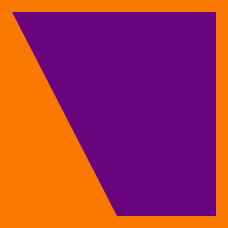Geometry

# Properties of Parallelograms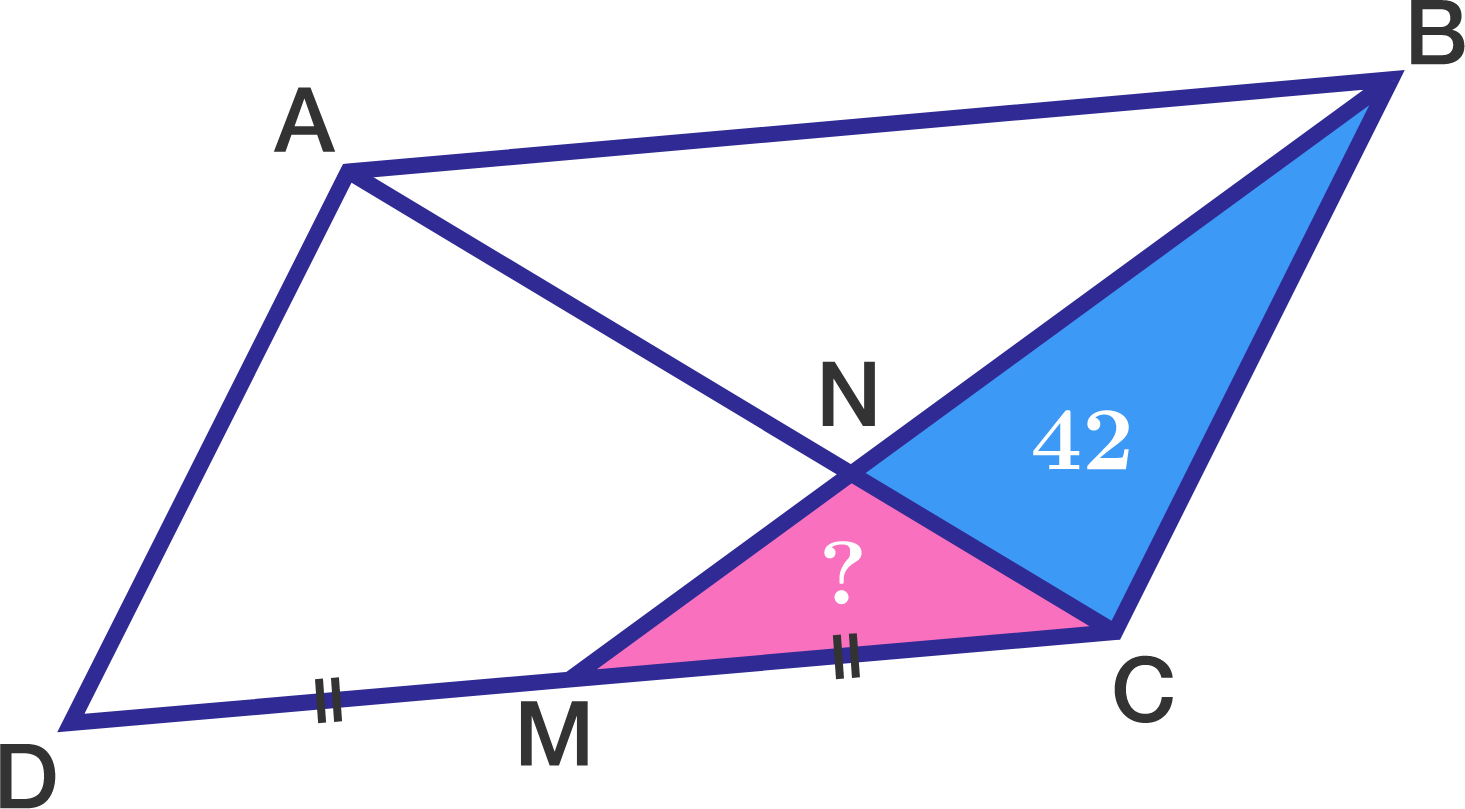In the diagram to the right, quadrilateral $ABCD$ is a parallelogram and $M$ is the midpoint of $\overline{DC}.$

If the area of $\triangle BNC$ is $42,$ what is the area of $\triangle MNC?$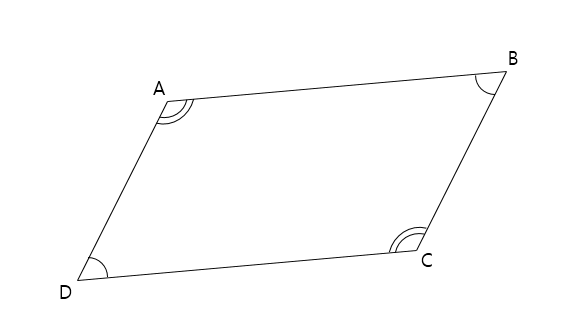In the above diagram, $\angle BAD$ = $\angle DCB$ and $\angle ADC$ = $\angle CBA .$ If the length of $\overline{AB}$ is $21$ and the length of $\overline{AD}$ is $16,$ what is the length of $\overline{BC}$?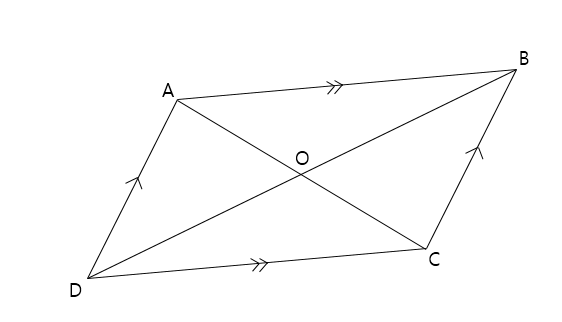In the quadrilateral $ABCD$ above, $\overline{AB}$ is parallel to $\overline{DC}$ and $\overline{AD}$ is parallel to $\overline{BC}.$ If the length of $\overline{DO}$= $41,$ then what is the length of $\overline{BO}?$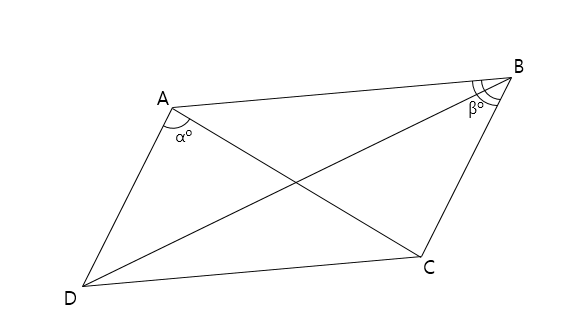In the above diagram, quadrilateral $ABCD$ is a parallelogram. If $\alpha = 69 ^\circ$ and $\beta = 55 ^\circ ,$ what is $\angle BAC$?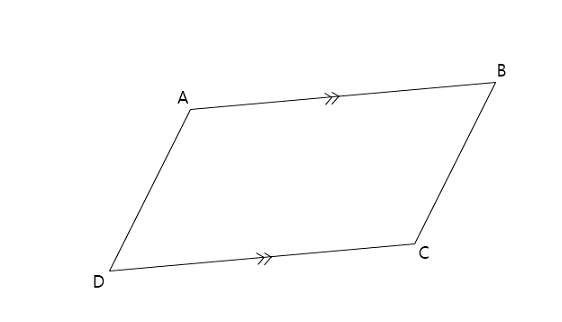In the quadrilateral $ABCD$ above, let $\lvert\overline{AB} \rvert$ denote the length of $\overline{AB}.$ If $\overline{AB}$ is parallel to $\overline{DC},$ $\lvert\overline{AB}\rvert=\lvert\overline{DC}\rvert = 23,$ and $\lvert\overline{AD}\rvert=15,$ what is $\lvert\overline{BC}\rvert?$

×